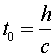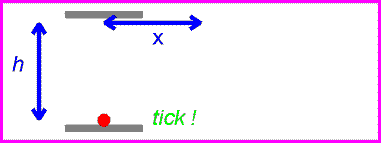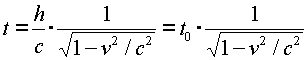Time dilation The fact that the speed of light is the same in all reference frames has the consequence that moving clocks run slow. This means that if two events occur at the same place, such as the ticks of a clock, a moving observer will measure the time between the events to be longer. The relation between a time measured by a stationary observer t0 to the time t measured by an observer moving with velocity v is:The gamma factor is common in relativity, and we will use it often. It is always greater than unity. If the velocity were greater than c, it would be undefined. This result can be shown to result from the fundamental postulate by considering a light clock. This imaginary clock would work by ticking every time a light pulse reflected back to the lower mirror as shown below.The time between ticks and tocks for the stationary clock is:One can now view the same clock as it moves past with velocity v.The time between ticks and tocks is again given by the distance traveled by the red pulse divided by c.Solving the above equation for t in terms of h, v and c givesThus the moving observer sees a longer time by the factor g defined in the previous page.